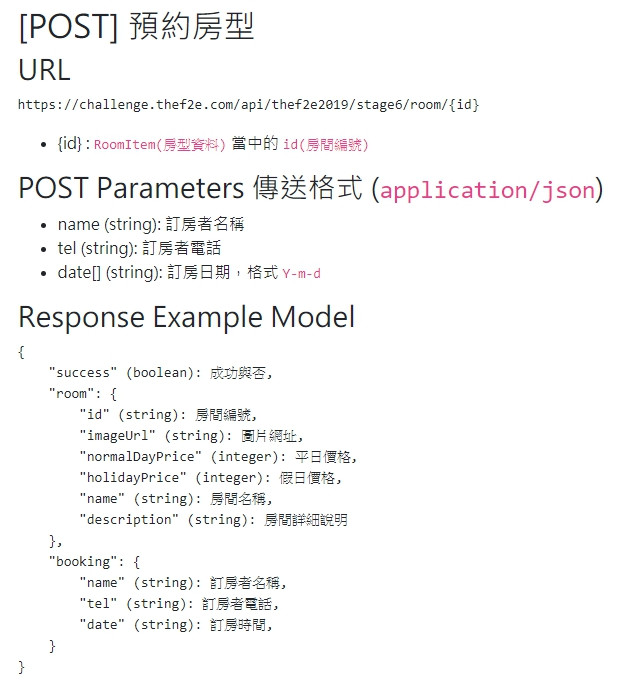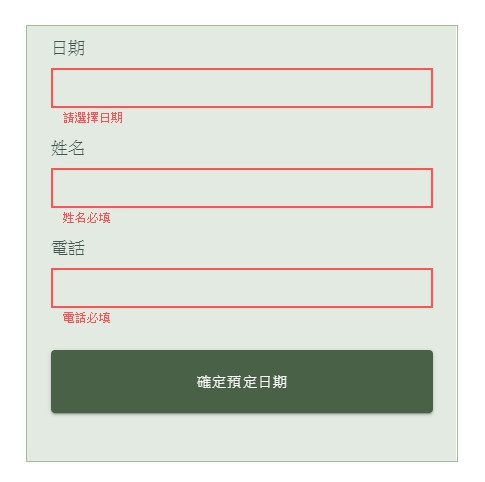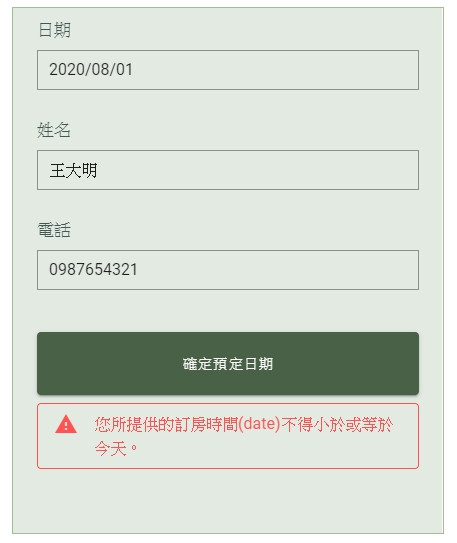#0

## [鼠年全馬] W35 - Vue出一個旅館預約平台(9)#### #呼叫API方式

• name (string): 訂房者名稱
• tel (string): 訂房者電話
• date[] (string): 訂房日期，格式 Y-m-d

API回傳結果包含

• 是否成功
• 房間詳細資訊

#### #Step 1

``````<v-text-field
outlined
dense
color="#a5bb94"
v-model="name"
required
:rules="[(v) => !!v || '姓名必填']"
></v-text-field>
``````#### #Step 2

``````<v-form ref="form">
//表單內容
</v-form>
``````

``````<v-btn
color="#496146"
dark
block
height="63"
class="mt-4"
@click="submit"
>確定預定日期
</v-btn>
``````

``````methods: {
submit() {
this.\$refs.form.validate();
},
},
``````

#### #Step 3

``````submit() {
if (this.\$refs.form.validate()) {
//表單驗證通過之後要做的事
}
},
``````

``````if (this.\$refs.form.validate()) {
this.\$http({
method: "POST",
Accept: "application/json",
/* 注意這裡要用你的token */
Authorization: "Bearer 你的token",
"Content-Type": "application/json",
},
/* 注意這裡要加上要預約的房間編號 */
url: `https://challenge.thef2e.com/api/thef2e2019/stage6/room/\${this.roomid}`,
data: JSON.stringify({
name: this.name,
tel: this.phone,
date: this.dates,
}),
})
.then((res) => {
//成功後要做的事
})
.catch((err) => {
//錯誤時要做的事
});
}
``````

#### #Step 4``````<v-alert v-if="alertmsg" class="mt-2" dense outlined type="error">
``````

data 的部分也要加上:

``````data: () => ({
...,
}),
``````

`catch()` 方法中把接收到的錯誤訊息放進 alertmsg:

``````.catch((err) => {
});
``````